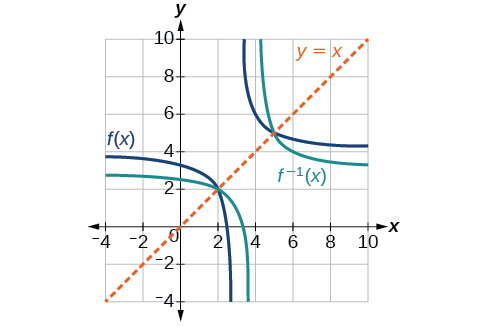# 1.7 Inverse functions  (Page 6/10)

 Page 6 / 10

Draw graphs of the functions and from [link] .Is there any function that is equal to its own inverse?

Yes. If $\text{\hspace{0.17em}}f={f}^{-1},\text{\hspace{0.17em}}$ then $\text{\hspace{0.17em}}f\left(f\left(x\right)\right)=x,\text{\hspace{0.17em}}$ and we can think of several functions that have this property. The identity function does, and so does the reciprocal function, because

$\frac{1}{\frac{1}{x}}=x$

Any function $\text{\hspace{0.17em}}f\left(x\right)=c-x,\text{\hspace{0.17em}}$ where $\text{\hspace{0.17em}}c\text{\hspace{0.17em}}$ is a constant, is also equal to its own inverse.

Access these online resources for additional instruction and practice with inverse functions.

Visit this website for additional practice questions from Learningpod.

## Key concepts

• If $\text{\hspace{0.17em}}g\left(x\right)\text{\hspace{0.17em}}$ is the inverse of $\text{\hspace{0.17em}}f\left(x\right),\text{\hspace{0.17em}}$ then $\text{\hspace{0.17em}}g\left(f\left(x\right)\right)=f\left(g\left(x\right)\right)=x.\text{\hspace{0.17em}}$ See [link] , [link] , and [link] .
• Each of the toolkit functions has an inverse. See [link] .
• For a function to have an inverse, it must be one-to-one (pass the horizontal line test).
• A function that is not one-to-one over its entire domain may be one-to-one on part of its domain.
• For a tabular function, exchange the input and output rows to obtain the inverse. See [link] .
• The inverse of a function can be determined at specific points on its graph. See [link] .
• To find the inverse of a formula, solve the equation $\text{\hspace{0.17em}}y=f\left(x\right)\text{\hspace{0.17em}}$ for $\text{\hspace{0.17em}}x\text{\hspace{0.17em}}$ as a function of $\text{\hspace{0.17em}}y.\text{\hspace{0.17em}}$ Then exchange the labels $\text{\hspace{0.17em}}x\text{\hspace{0.17em}}$ and $\text{\hspace{0.17em}}\text{\hspace{0.17em}}y.\text{\hspace{0.17em}}\text{\hspace{0.17em}}$ See [link] , [link] , and [link] .
• The graph of an inverse function is the reflection of the graph of the original function across the line $\text{\hspace{0.17em}}y=x.\text{\hspace{0.17em}}$ See [link] .

## Verbal

Describe why the horizontal line test is an effective way to determine whether a function is one-to-one?

Each output of a function must have exactly one output for the function to be one-to-one. If any horizontal line crosses the graph of a function more than once, that means that $\text{\hspace{0.17em}}y$ -values repeat and the function is not one-to-one. If no horizontal line crosses the graph of the function more than once, then no $\text{\hspace{0.17em}}y$ -values repeat and the function is one-to-one.

Why do we restrict the domain of the function $\text{\hspace{0.17em}}f\left(x\right)={x}^{2}\text{\hspace{0.17em}}$ to find the function’s inverse?

Can a function be its own inverse? Explain.

Yes. For example, $\text{\hspace{0.17em}}f\left(x\right)=\frac{1}{x}\text{\hspace{0.17em}}$ is its own inverse.

Are one-to-one functions either always increasing or always decreasing? Why or why not?

How do you find the inverse of a function algebraically?

Given a function $\text{\hspace{0.17em}}y=f\left(x\right),\text{\hspace{0.17em}}$ solve for $\text{\hspace{0.17em}}x\text{\hspace{0.17em}}$ in terms of $\text{\hspace{0.17em}}y.\text{\hspace{0.17em}}$ Interchange the $\text{\hspace{0.17em}}x\text{\hspace{0.17em}}$ and $\text{\hspace{0.17em}}y.\text{\hspace{0.17em}}$ Solve the new equation for $\text{\hspace{0.17em}}y.\text{\hspace{0.17em}}$ The expression for $\text{\hspace{0.17em}}y\text{\hspace{0.17em}}$ is the inverse, $\text{\hspace{0.17em}}y={f}^{-1}\left(x\right).$

## Algebraic

Show that the function $\text{\hspace{0.17em}}f\left(x\right)=a-x\text{\hspace{0.17em}}$ is its own inverse for all real numbers $\text{\hspace{0.17em}}a.\text{\hspace{0.17em}}$

For the following exercises, find $\text{\hspace{0.17em}}{f}^{-1}\left(x\right)\text{\hspace{0.17em}}$ for each function.

$f\left(x\right)=x+3$

${f}^{-1}\left(x\right)=x-3$

$f\left(x\right)=x+5$

$f\left(x\right)=2-x$

${f}^{-1}\left(x\right)=2-x$

$f\left(x\right)=3-x$

$f\left(x\right)=\frac{x}{x+2}$

${f}^{-1}\left(x\right)=\frac{-2x}{x-1}$

$f\left(x\right)=\frac{2x+3}{5x+4}$

For the following exercises, find a domain on which each function $\text{\hspace{0.17em}}f\text{\hspace{0.17em}}$ is one-to-one and non-decreasing. Write the domain in interval notation. Then find the inverse of $\text{\hspace{0.17em}}f\text{\hspace{0.17em}}$ restricted to that domain.

$f\left(x\right)={\left(x+7\right)}^{2}$

domain of $f\left(x\right):\text{\hspace{0.17em}}\left[-7,\infty \right);\text{\hspace{0.17em}}{f}^{-1}\left(x\right)=\sqrt{x}-7$

$f\left(x\right)={\left(x-6\right)}^{2}$

$f\left(x\right)={x}^{2}-5$

domain of $\text{\hspace{0.17em}}f\left(x\right):\text{\hspace{0.17em}}\left[0,\infty \right);\text{\hspace{0.17em}}{f}^{-1}\left(x\right)=\sqrt{x+5}$

Given $\text{\hspace{0.17em}}f\left(x\right)=\frac{x}{2}+x\text{\hspace{0.17em}}$ and $\text{\hspace{0.17em}}g\left(x\right)=\frac{2x}{1-x}:$

1. Find $\text{\hspace{0.17em}}f\left(g\left(x\right)\right)\text{\hspace{0.17em}}$ and $\text{\hspace{0.17em}}g\left(f\left(x\right)\right).$
2. What does the answer tell us about the relationship between $\text{\hspace{0.17em}}f\left(x\right)\text{\hspace{0.17em}}$ and $\text{\hspace{0.17em}}g\left(x\right)?$

a. and $\text{\hspace{0.17em}}g\left(f\left(x\right)\right)=x.\text{\hspace{0.17em}}$ b. This tells us that $\text{\hspace{0.17em}}f\text{\hspace{0.17em}}$ and $\text{\hspace{0.17em}}g\text{\hspace{0.17em}}$ are inverse functions

#### Questions & Answers

how fast can i understand functions without much difficulty
Joe Reply
what is set?
Kelvin Reply
a colony of bacteria is growing exponentially doubling in size every 100 minutes. how much minutes will it take for the colony of bacteria to triple in size
Divya Reply
I got 300 minutes. is it right?
Patience
no. should be about 150 minutes.
Jason
It should be 158.5 minutes.
Mr
ok, thanks
Patience
100•3=300 300=50•2^x 6=2^x x=log_2(6) =2.5849625 so, 300=50•2^2.5849625 and, so, the # of bacteria will double every (100•2.5849625) = 258.49625 minutes
Thomas
what is the importance knowing the graph of circular functions?
Arabella Reply
can get some help basic precalculus
ismail Reply
What do you need help with?
Andrew
how to convert general to standard form with not perfect trinomial
Camalia Reply
can get some help inverse function
ismail
Rectangle coordinate
Asma Reply
how to find for x
Jhon Reply
it depends on the equation
Robert
yeah, it does. why do we attempt to gain all of them one side or the other?
Melissa
whats a domain
mike Reply
The domain of a function is the set of all input on which the function is defined. For example all real numbers are the Domain of any Polynomial function.
Spiro
Spiro; thanks for putting it out there like that, 😁
Melissa
foci (–7,–17) and (–7,17), the absolute value of the differenceof the distances of any point from the foci is 24.
Churlene Reply
difference between calculus and pre calculus?
Asma Reply
give me an example of a problem so that I can practice answering
Jenefa Reply
x³+y³+z³=42
Robert
dont forget the cube in each variable ;)
Robert
of she solves that, well ... then she has a lot of computational force under her command ....
Walter
what is a function?
CJ Reply
I want to learn about the law of exponent
Quera Reply
explain this
Hinderson Reply

### Read also:

#### Get Jobilize Job Search Mobile App in your pocket Now!

Source:  OpenStax, Precalculus. OpenStax CNX. Jan 19, 2016 Download for free at https://legacy.cnx.org/content/col11667/1.6
Google Play and the Google Play logo are trademarks of Google Inc.

Notification Switch

Would you like to follow the 'Precalculus' conversation and receive update notifications?ByByBy Hannah ShethBy Sarah WarrenBy Subramanian DivyaBy Anonymous UserBy OpenStaxBy Madison ChristianByBy Madison ChristianBy OpenStaxBy OpenStax